Search by Topic

Resources tagged with Coordinates similar to Arranging Cubes:

Filter by: Content type:
Age range:
Challenge level:

There are 21 results

Broad Topics > Coordinates and Coordinate Geometry > CoordinatesFred the Class Robot

Age 7 to 11 Challenge Level:

Billy's class had a robot called Fred who could draw with chalk held underneath him. What shapes did the pupils make Fred draw?Eight Hidden Squares

Age 7 to 14 Challenge Level:

On the graph there are 28 marked points. These points all mark the vertices (corners) of eight hidden squares. Can you find the eight hidden squares?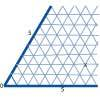Cartesian Isometric

Age 7 to 11 Challenge Level:

The graph below is an oblique coordinate system based on 60 degree angles. It was drawn on isometric paper. What kinds of triangles do these points form?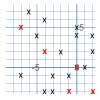Ten Hidden Squares

Age 7 to 11 Challenge Level:

These points all mark the vertices (corners) of ten hidden squares. Can you find the 10 hidden squares?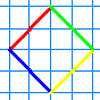A Cartesian Puzzle

Age 7 to 11 Challenge Level:

Find the missing coordinates which will form these eight quadrilaterals. These coordinates themselves will then form a shape with rotational and line symmetry.Cops and Robbers

Age 7 to 16 Challenge Level:

Can you find a reliable strategy for choosing coordinates that will locate the robber in the minimum number of guesses?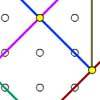Coordinate Tan

Age 7 to 11 Challenge Level:

What are the coordinates of the coloured dots that mark out the tangram? Try changing the position of the origin. What happens to the coordinates now?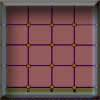Criss Cross Quiz

Age 7 to 11 Challenge Level:

A game for 2 players. Practises subtraction or other maths operations knowledge.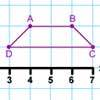Transformation Tease

Age 7 to 11 Challenge Level:

What are the coordinates of this shape after it has been transformed in the ways described? Compare these with the original coordinates. What do you notice about the numbers?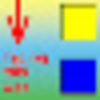Coordinating Classroom Coordinates

Age 7 to 11

This article describes a practical approach to enhance the teaching and learning of coordinates.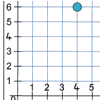Two Number Lines

Age 7 to 11 Challenge Level:

Max and Mandy put their number lines together to make a graph. How far had each of them moved along and up from 0 to get the counter to the place marked?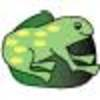The Lily Pond

Age 7 to 11 Challenge Level:

Freddie Frog visits as many of the leaves as he can on the way to see Sammy Snail but only visits each lily leaf once. Which is the best way for him to go?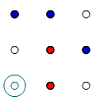Coordinate Cunning

Age 7 to 11 Challenge Level:

A game for two people that can be played with pencils and paper. Combine your knowledge of coordinates with some strategic thinking.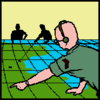Going Places with Mathematicians

Age 7 to 14

This article looks at the importance in mathematics of representing places and spaces mathematics. Many famous mathematicians have spent time working on problems that involve moving and mapping. . . .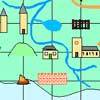Treasure Island

Age 7 to 11 Challenge Level:

Write down what you can see at the coordinates of the treasure island map. The words can be used in a special way to find the buried treasure. Can you work out where it is?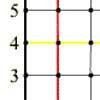What Are You Plotting?

Age 7 to 11 Challenge Level:

Investigate the positions of points which have particular x and y coordinates. What do you notice?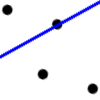Dotty Relationship

Age 7 to 11 Challenge Level:

Can you draw perpendicular lines without using a protractor? Investigate how this is possible.Being Curious - Primary Geometry

Age 5 to 11 Challenge Level:

Geometry problems for inquiring primary learners.Being Collaborative - Primary Geometry

Age 5 to 11 Challenge Level:

Geometry problems for primary learners to work on with others.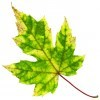Being Resourceful - Primary Geometry

Age 5 to 11 Challenge Level:

Geometry problems at primary level that require careful consideration.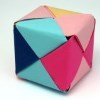Being Resilient - Primary Geometry

Age 5 to 11 Challenge Level:

Geometry problems at primary level that may require resilience.